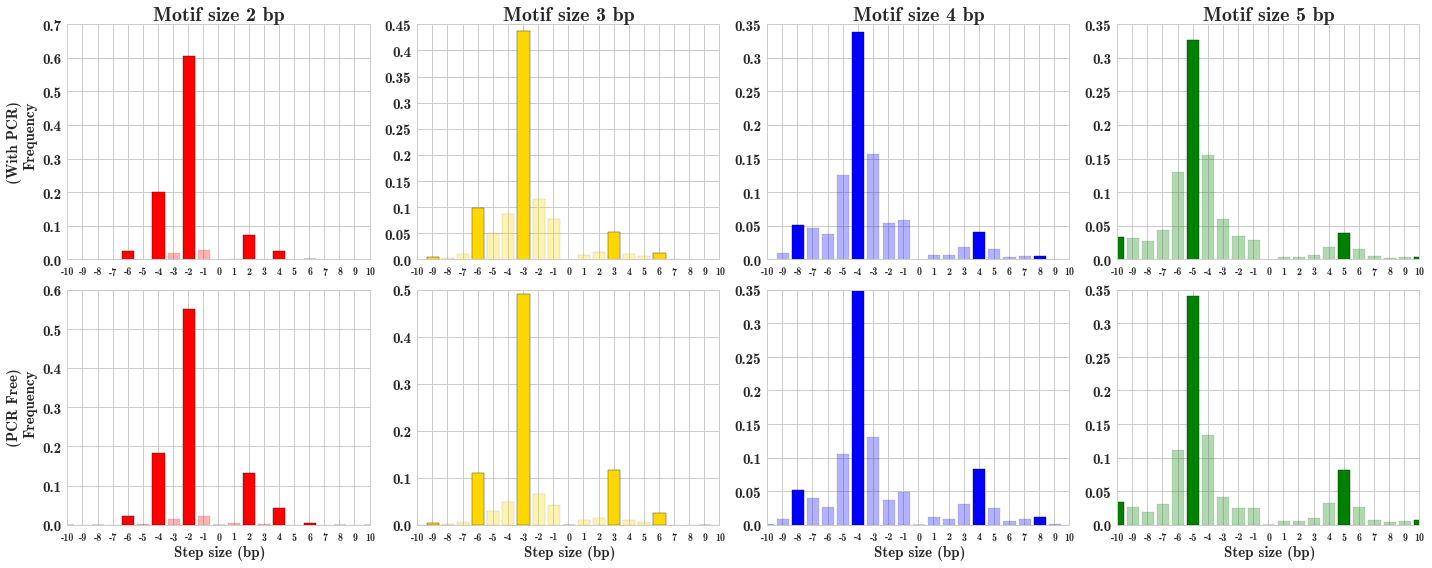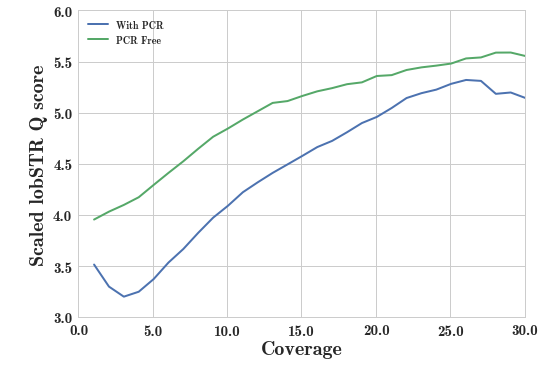In :
import IPython.core.display
import math
import pandas as pd
import seaborn as sns
import sys
sys.path.append("/home/mgymrek/workspace/lobstr-code/experimental")
import visualize_stutter

BTEAM_NOISE_MODEL_PREFIX = "/home/mgymrek/workspace/mgymrek.github.io/data/BTeam_PCR"
CTEAM_NOISE_MODEL_PREFIX = "/home/mgymrek/workspace/mgymrek.github.io/data/CTeam_PCRfree"

BTEAM_SCORES_FILE = "/home/mgymrek/workspace/mgymrek.github.io/data/bteam_scores.tab"
CTEAM_SCORES_FILE = "/home/mgymrek/workspace/mgymrek.github.io/data/cteam_scores.tab"

This post was made using IPython notebook. The source (for a slightly earlier draft) is on github.

## Introduction

Recently, Illumina came out with the TruSeq PCR free protocol for whole genome sequencing. They claim on the website that the big benefits of this method are "reduced library bias and gaps" leading to "unsurpassed data quality". In practice, the PCR free genomes coming out (e.g. Platinum Genomes and the PCR-free 250bp read trios from the 1000 Genomes Project) do seem to be of excellent quality.

Our lab is very interested in genotyping short tandem repeats (STRs) from whole genome sequencing datasets. For us, the idea of PCR free libraries is extremely exciting, since it has the potential to greatly reduce one of our major sources of errors: stutter noise. Noise at STRs is thought to result from polymerase slippage during amplification, and results in many reads being off from the true allele by a unit (or more than one unit) of the repeat motif. Below are some example alignments where some reads are a result of stutter noise (ok ok, I also put these here as an excuse to try out/show off the new "export figure" feature of my pybamview tool, which you should check out if you often find yourself looking at sequence alignments for indels).

In :
IPython.core.display.SVG(url="http://web.mit.edu/~mgymrek/Public/images/pybamview_chrY_3131127_3131200.svg")

Out:
In :
IPython.core.display.SVG(url="http://web.mit.edu/~mgymrek/Public/images/pybamview_chrY_4075820_4075900.svg")

Out:

The examples above are from male chrX calls, so there is only one true allele present. In the first example, one read is off by one repeat unit from the true allele (it is missing an AGAT). In the second, there's an example of a stutter read off by 2 repeat units. We see examples such as these quite commonly. It is even worse at diploid loci where there are potentially 2 alleles, plus stutter, making it difficult to determine the true genotype. In our program lobSTR, we attempt to model this noise when scoring genotypes. But in low coverage data, such as the 1000 Genomes Project samples, where we often have only a single read for each call, the model's not going to help us much. If you have one read, and that read is from stutter, you're almost certainly going to make a wrong call at that locus.

The PCR-free method has the potential to dramatically reduce the PCR stutter problem at STRs. Below I compare two whole genome sequencing datasets of similar quality, one PCR free and one not, and show evidence that the PCR free protocol cleans up a lot of the noise at STRs, reducing the stutter rate by several fold.

## Datasets and STR genotyping

Here I compare stutter noise in two whole genome sequencing datasets from HGDP samples:
• HGDP00542 (with PCR): This dataset was obtained from the CEPH website and can be downloaded here. This is a Papuan individual sequenced to 25.9x. The dataset was published with the Meyer et al. Denisova paper in Science 2012.
• High coverage PCR free dataset (This dataset is not yet published). This is another HGDP sample sequenced to 37.6x coverage using the PCR-free protocol.

Both datasets consist of 100bp paired end reads for male samples. Besides the difference in the PCR free protocol vs. not, these datasets are expected to have similar quality.

I used our STR genotyping tool, lobSTR to genotype STRs in these samples. For alignment, I used v2.0.4. For allelotyping, I used v2.0.5. For anyone that wants all the gory details the specific commands I used are at the bottom of this post.

For each sample, I looked at loci on chrX to estimate how many reads are the result of stutter noise. I took all loci with at least 5x coverage and a unique modal allele (the allele supported by the most reads) which I called the true allele. Since the samples are male, chrX calls should be hemizygous, and any read not matching the true genotype is from stutter (or an alignment error, which definitely happens, but I'm ignoring that here). This is the same method lobSTR uses to train its stutter model.

The first metric I looked at was simply the percentage of reads determined to be from stutter noise. Below are the results broken up by motif size. The stutter rate clearly decreases with motif length (homopolymers are by far the worst, and the bane of anyone's existence who works with STR calling, whereas longer motifs have very few reads from stutter). On average, the PCR free dataset had a stutter rate more than 4-fold lower than the dataset using the non-PCR-free protocol! Pretty encouraging.

Motif length Stutter prob. - with PCR Stutter prob. - PCR free Fold decrease
1 0.17 0.027 6.30
2 0.038 0.011 3.45
3 0.011 0.0029 3.79
4 0.0069 0.0016 4.31
5 0.0074 0.0015 4.93
6 0.014 0.0010 14.0
Average 0.051 0.011 4.63

While the rate of stutter reads was greatly reduced in the PCR-free dataset, the distribution of stutter error sizes was about the same. Below is the distribution of error sizes for each motif length (I only showed periods 2-5 to save space, but the plots for periods 1 and 6 are similar). You can see what we have observed many times: stutter noise tends to come in multiples of the repeat unit length and has a strong bias toward decreasing the number of repeats from the true allele. This is true for both datasets, perhaps with the PCR-free dataset having a slightly lower bias toward decreasing allele length.

In :
sns.set(style="whitegrid")
bteam_stepmodel = visualize_stutter.StepModel(BTEAM_NOISE_MODEL_PREFIX + ".stepmodel")
cteam_stepmodel = visualize_stutter.StepModel(CTEAM_NOISE_MODEL_PREFIX + ".stepmodel")

# Plot 6x2 grid of period x pcr free/not
fig = plt.figure()
fig.set_size_inches((15, 6))
for period in range(2, 6):
if period == 2: yl = "(With PCR)\nFrequency"
else: yl = None
bteam_stepmodel.PlotHistogram(period, ax=ax, ylab=yl, title="Motif size %s bp"%period, xlim=[-10,10])
if period == 2: yl = "(PCR Free)\nFrequency"
else: yl = None
cteam_stepmodel.PlotHistogram(period, ax=ax, xlab="Step size (bp)", ylab=yl, xlim=[-10,10])
fig.tight_layout()In :
bscores = pd.read_csv(BTEAM_SCORES_FILE, sep="\t", names=["chrom","start","coverage_b","score_b"])
scores = pd.merge(bscores, cscores, on=["chrom","start"]) # only take loci covered in both

In :
scores.ix[scores["score_b"]==1,"score_b"] = 0.999999 # can't have 1 for log
scores.ix[scores["score_c"]==1,"score_c"] = 0.999999

scores["logscore_b"] = scores["score_b"].apply(lambda x: -1*math.log10(1-x))
scores["logscore_c"] = scores["score_c"].apply(lambda x: -1*math.log10(1-x))

# mean score by cov
score_bycov_b = scores.groupby("coverage_b", as_index=False).agg({"logscore_b": np.mean})
score_bycov_c = scores.groupby("coverage_c", as_index=False).agg({"logscore_c": np.mean})
score_bycov_b.columns = ["coverage","logscore_b"]
score_bycov_c.columns = ["coverage","logscore_c"]
score_bycov = pd.merge(score_bycov_b, score_bycov_c)
score_bycov = score_bycov[score_bycov["coverage"]<=30]


Another metric I looked at was the quality score of STR calls. For each genotype, lobSTR reports a quality score. This score is calculated as:

In :
%%latex
\begin{equation}
Q = \frac{L_{ML}}{\sum_{g \in G}L_g}
\end{equation}

\begin{equation} Q = \frac{L_{ML}}{\sum_{g \in G}L_g} \end{equation}
where $L_{ML}$ is the likelihood of the maximum liklihood genotype, and $G$ is the set of all possible diploid genotypes, and $L_g$ is the likelihood of genotype $g$.

A $Q$ value close to 1 means a quite confident call, whereas a $Q$ close to 0 means the call is not much better than the other possible genotype calls.

I compared the average $Q$ for different coverage levels in the two datasets. The y-axis gives $-1\log10(1-Q)$ (higher=better). The scores are significantly higher at all coverage levels in the PCR-free dataset:

In :
fig = plt.figure()
ax.plot(score_bycov["coverage"], score_bycov["logscore_b"], lw=2, label="With PCR")
ax.plot(score_bycov["coverage"], score_bycov["logscore_c"], lw=2, label="PCR Free");
ax.set_xlabel("Coverage", size=20)
ax.set_ylabel("Scaled lobSTR Q score", size=20)
ax.set_xticklabels(ax.get_xticks(), size=15)
ax.set_yticklabels(ax.get_yticks(), size=15);
ax.legend(loc="upper left");## Conclusion

In conclusion, the Illumina PCR-free protocol seems to have great promise for improving STR calls from high throughput sequencing datasets. While I only compared two datasets here, we see similar trends in other datasets: PCR free samples have much lower stutter noise at STRs. Of particular note is the improvement at homopolymers, which have until now been nearly impossible to call with any reasonable accuracy. This holds great promise for genotyping STRs as more and more sequencing datasets are generating using this protocol.